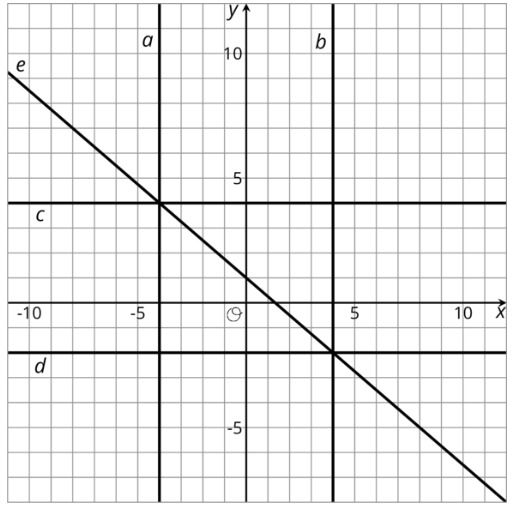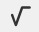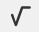PSABPW6W - Cool-Down---Acc7.5 Lesson 9 Slopes and Equations for All Kinds of Lines (8.EE.B.6)
Part A)

Here are 5 lines on a coordinate grid:Write equations for lines a,b,c,d, and e.

Write the equation for line a:

Write the equation using the "WIRIS editor" buttonPart B)

Write the equation for line b:

Write the equation using the "WIRIS editor" buttonPart C)

Write the equation for line c:

Write the equation using the "WIRIS editor" buttonPart D)

Write the equation for line d:

Write the equation using the "WIRIS editor" buttonWrite the equation using the "WIRIS editor" button